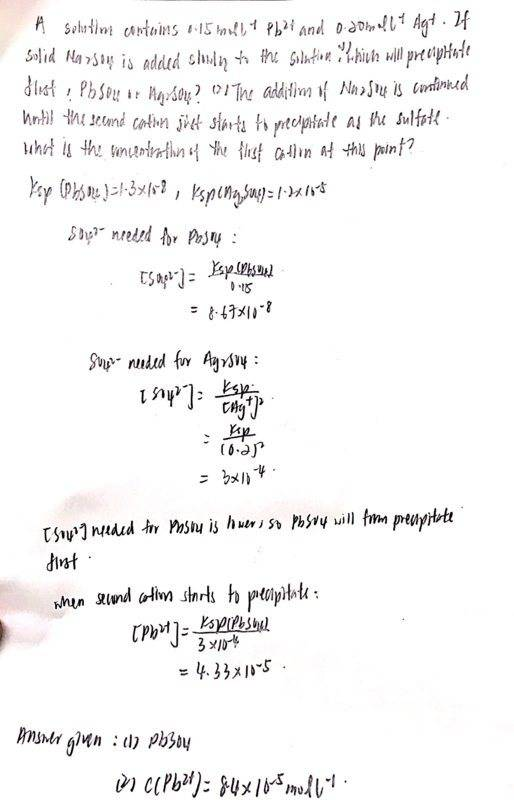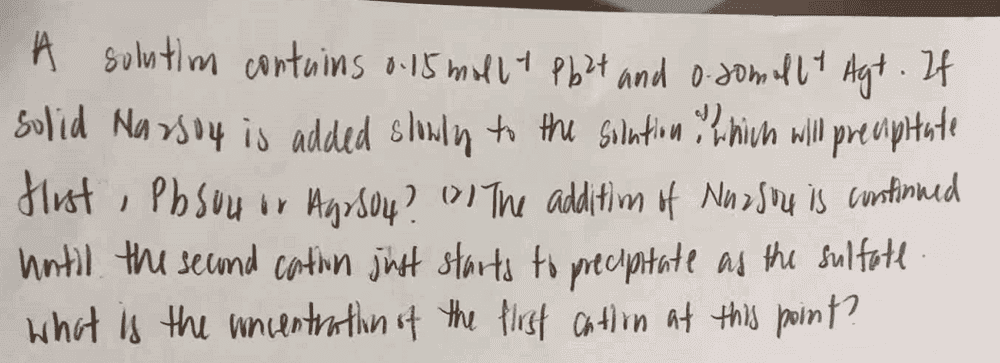# Equilibrio of a sparingly soluble ionic compound

• Chemistry
daphnelee-mh
Homework Statement:
Calculate the concentration of the first cation until second cation just starts to precipitate as sulfate
Relevant Equations:
Ksp
I got the first question correct but I don’t understand the answer given for second question which is c (Pb^2+) = 8.4x10^-5 molL ^-1. It divided the concentrate for sulphate ion of Ag2SO4 into 2 , but it is the solubility right ?#### Attachments

Mentor
This is very difficult to readIt divided the concentrate for sulphate ion of Ag2SO4 into 2

As far as I can tell - it didn't.

daphnelee-mhMy answer is 4.33 x 10^-5, but answer given is 8.4x10^-5, how can I get it?

Mentor
Next time please type the answer (takes less time than writing and taking pictures).

As far as I can tell answer given is wrong, your approach looks OK.

daphnelee-mh
Sorry, my computer have problems that time, I can just upload using phone. Therefore, is it the given answer is wrong?

Mentor
Looks like, I got the same answer you did.

•daphnelee-mh